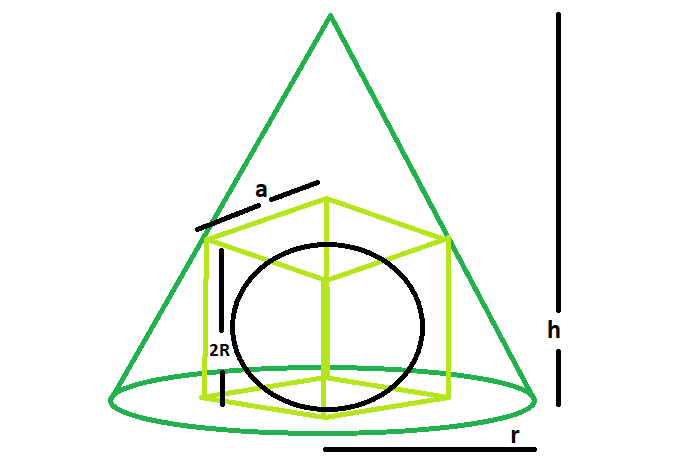# Largest sphere that can be inscribed within a cube which is in turn inscribed within a right circular cone

Given here is a right circular cone of radius r and perpendicular height h, which is inscribed in a cube which in turn is inscribed in a sphere, the task is to find the radius of the sphere.

Examples:

```Input: h = 5, r = 6
Output: 1.57306

Input: h = 8, r = 11
Output: 2.64156
```## Recommended: Please try your approach on {IDE} first, before moving on to the solution.

Approach:

Below is the implementation of the above approach:

## C++

 `// C++ Program to find the biggest sphere ` `// which is inscribed within a cube which in turn ` `// inscribed within a right circular cone ` ` `  `#include ` `using` `namespace` `std; ` ` `  `// Function to find the radius of the sphere ` `float` `sphereSide(``float` `h, ``float` `r) ` `{ ` `    ``// height and radius cannot be negative ` `    ``if` `(h < 0 && r < 0) ` `        ``return` `-1; ` ` `  `    ``// radius of the sphere ` `    ``float` `R = ((h * r * ``sqrt``(2)) / (h + ``sqrt``(2) * r)) / 2; ` ` `  `    ``return` `R; ` `} ` ` `  `// Driver code ` `int` `main() ` `{ ` `    ``float` `h = 5, r = 6; ` ` `  `    ``cout << sphereSide(h, r) << endl; ` ` `  `    ``return` `0; ` `} `

## Java

 `// Java Program to find the biggest sphere ` `// which is inscribed within a cube which in turn ` `// inscribed within a right circular cone ` `import` `java.lang.Math; ` ` `  `class` `GFG ` `{ ` `     `  `// Function to find the radius of the sphere ` `static` `float` `sphereSide(``float` `h, ``float` `r) ` `{ ` `    ``// height and radius cannot be negative ` `    ``if` `(h < ``0` `&& r < ``0``) ` `        ``return` `-``1``; ` ` `  `    ``// radius of the sphere ` `    ``float` `R = (``float``)((h * r * Math.sqrt(``2``)) /  ` `                    ``(h + Math.sqrt(``2``) * r)) / ``2``; ` ` `  `    ``return` `R; ` `} ` ` `  `// Driver code ` `public` `static` `void` `main(String[] args) ` `{ ` `    ``float` `h = ``5``, r = ``6``; ` ` `  `    ``System.out.println(sphereSide(h, r)); ` ` `  `} ` `} ` ` `  `// This code is contributed by Code_Mech. `

## Python3

 `# Program to find the biggest sphere ` `# which is inscribed within a cube which in turn ` `# inscribed within a right circular cone ` `import` `math ` ` `  `# Function to find the radius of the sphere ` `def` `sphereSide(h, r): ` ` `  `    ``# height and radius cannot be negative ` `    ``if` `h < ``0` `and` `r < ``0``: ` `        ``return` `-``1` ` `  `    ``# radius of the sphere ` `    ``R ``=` `(((h ``*` `r ``*` `math.sqrt(``2``))) ``/`  `              ``(h ``+` `math.sqrt(``2``) ``*` `r) ``/` `2``) ` ` `  `    ``return` `R ` ` `  `# Driver code ` `h ``=` `5``; r ``=` `6` `print``(sphereSide(h, r)) ` ` `  `# This code is contributed by Shrikant13 `

## C#

 `// C# Program to find the biggest sphere ` `// which is inscribed within a cube which in turn ` `// inscribed within a right circular cone ` `using` `System; ` ` `  `class` `GFG ` `{ ` `     `  `// Function to find the radius of the sphere ` `static` `float` `sphereSide(``float` `h, ``float` `r) ` `{ ` `    ``// height and radius cannot be negative ` `    ``if` `(h < 0 && r < 0) ` `        ``return` `-1; ` ` `  `    ``// radius of the sphere ` `    ``float` `R = (``float``)((h * r * Math.Sqrt(2)) /  ` `                      ``(h + Math.Sqrt(2) * r)) / 2; ` ` `  `    ``return` `R; ` `} ` ` `  `// Driver code ` `public` `static` `void` `Main() ` `{ ` `    ``float` `h = 5, r = 6; ` ` `  `    ``Console.WriteLine(sphereSide(h, r)); ` `} ` `} ` ` `  `// This code is contributed by Code_Mech `

## PHP

 ` `

Output:

```1.57306
```

My Personal Notes arrow_drop_upCheck out this Author's contributed articles.

If you like GeeksforGeeks and would like to contribute, you can also write an article using contribute.geeksforgeeks.org or mail your article to contribute@geeksforgeeks.org. See your article appearing on the GeeksforGeeks main page and help other Geeks.

Please Improve this article if you find anything incorrect by clicking on the "Improve Article" button below.

Improved By : shrikanth13, Code_Mech